# 谷歌开源用于训练量子模型的机器学习框架 TensorFlow Quantum

• 2020-03-12
• 本文字数：6802 字

阅读完需：约 22 分钟2020 年 3 月 11 日，TensorFlow Dev Summit 峰会通过线上直播的方式与各位开发者见面。会上，谷歌介绍了近期宣布开源的一款用于训练量子模型的机器学习库 TensorFlow Quantum（简称 TFQ）。谷歌表示，该量子机器学习模型能够处理量子数据，并能够在量子计算机上执行。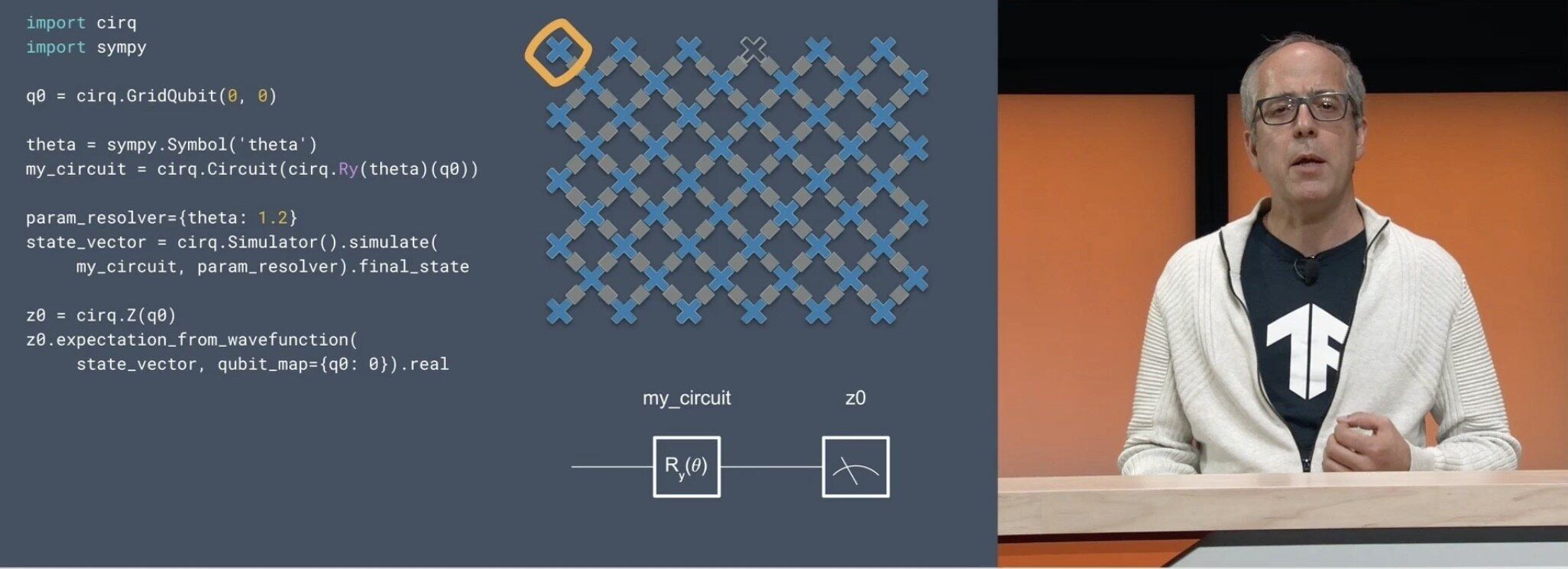## 量子机器学习模型是什么？

TFQ 包含了特定量子计算所需的基本结构，例如量子比特、门、电路和测量运算符。用户指定的量子计算然后可以在模拟或真实硬件上执行。Cirq 也包含了大量机器，可帮助用户为 NISQ 机器（例如编译器和调度程序）设计出高效算法，并且能使混合量子经典算法的实现在量子电路模拟器上运行，并最终在量子处理器上运行。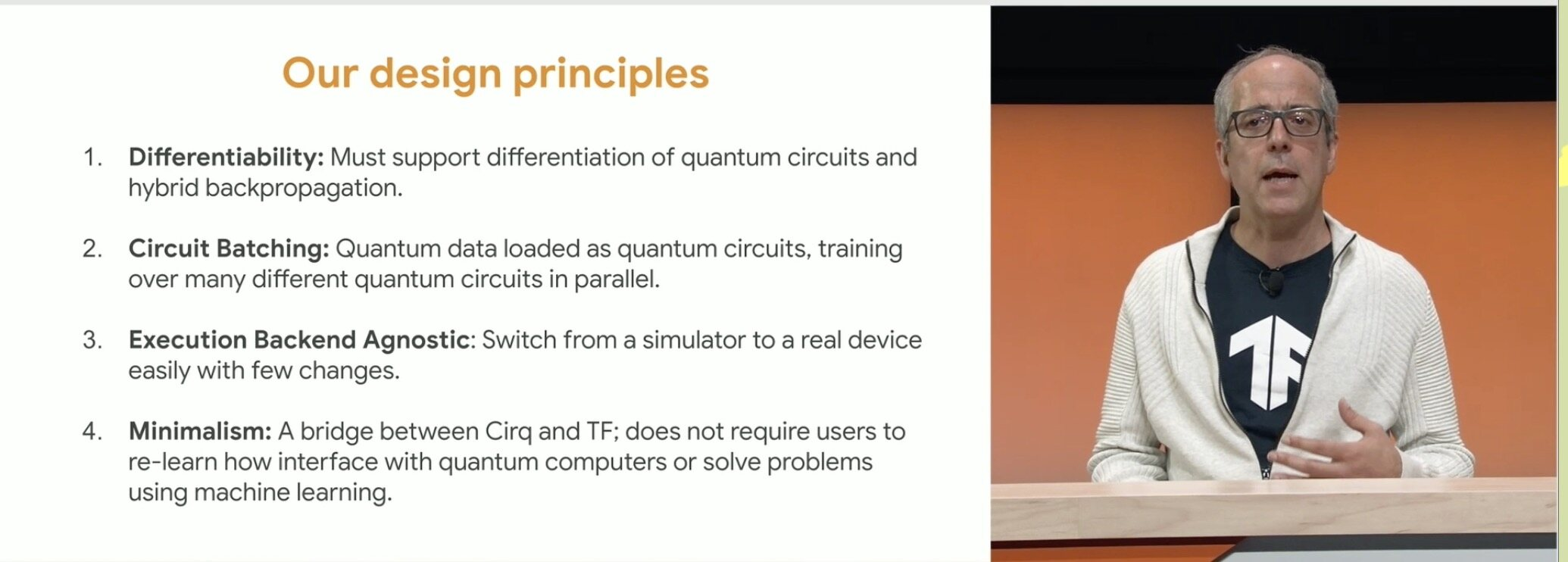## TFQ 的工作原理

TFQ 能够帮助研究人员在单个计算图中构建量子数据集、量子模型和作为张量的经典控制参数。导致经典概率事件的量子测量结果可通过 TensorFlow Ops 获得，可用标准 Keras 函数进行训练。

• 准备一个量子数据集

• 评估量子神经网络模型

• 样本或平均值

• 评估经典神经网络模型

• 评估成本函数

• 评估梯度和更新参数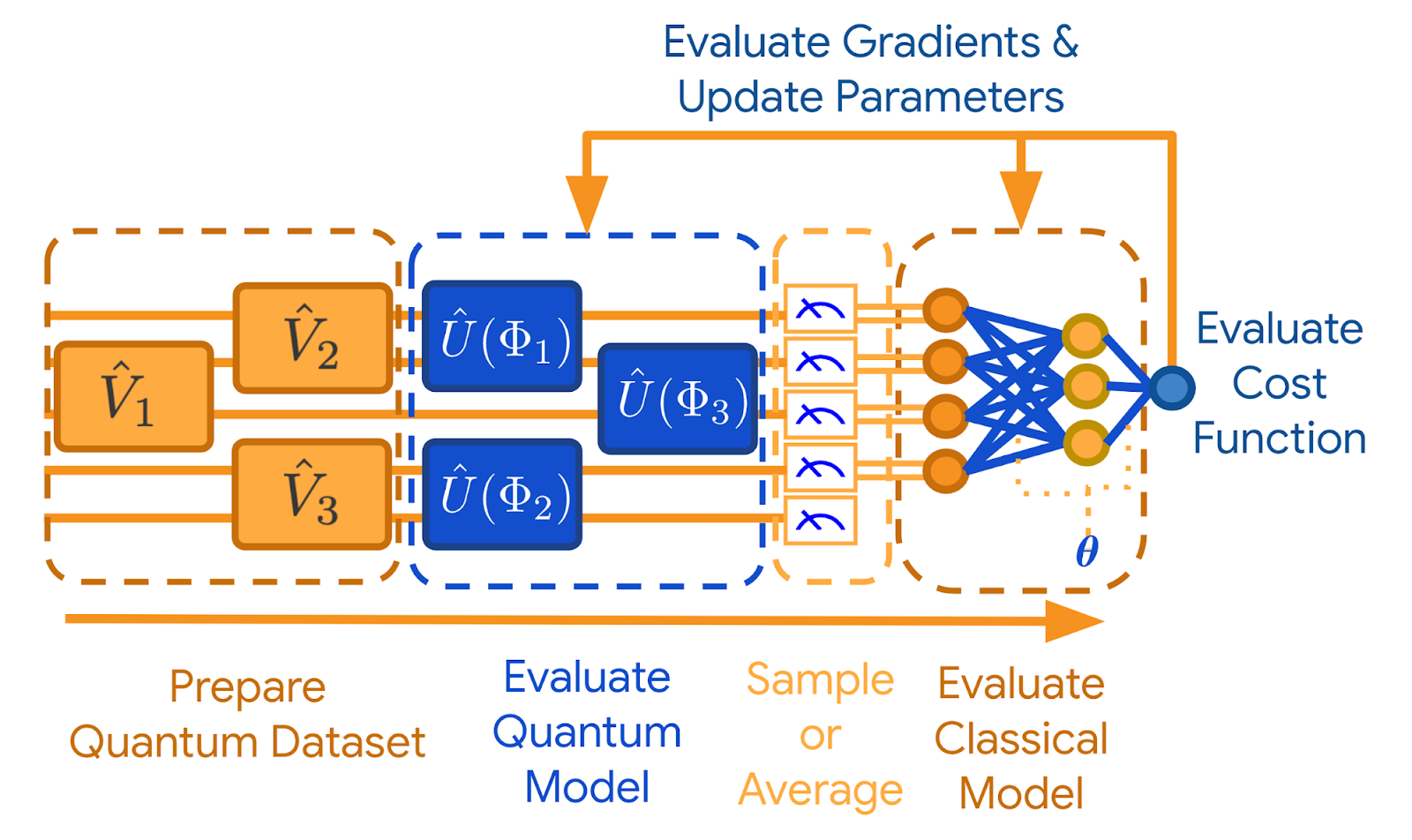TensorFlow Quantum 的关键特征是它能够同时训练和执行多个量子电路。TensorFlow 能够跨计算机集群进行并行计算，并能够在多核计算机上模拟相对较大的量子电路。为了实现后者，谷歌还宣布发布新的高性能开源量子电路模拟器 qsim (项目地址：https://github.com/quantumlib/qsim)，该模拟器已证明能够在 111 秒内模拟门深度为 14 的 32 比特量子电路。

## 如何使用 TensorFlow Quantum？

pip install -q tensorflow-quantum

import tensorflow as tfimport tensorflow_quantum as tfqimport cirqimport sympyimport numpy as np# visualization tools%matplotlib inlineimport matplotlib.pyplot as pltfrom cirq.contrib.svg import SVGCircuit

### 参数化量子电路

a, b = sympy.symbols('a b')

# Create two qubitsq0, q1 = cirq.GridQubit.rect(1, 2)# Create a circuit on these qubits using the parameters you created above.circuit = cirq.Circuit(    cirq.rx(a).on(q0),    cirq.ry(b).on(q1), cirq.CNOT(control=q0, target=q1))SVGCircuit(circuit)

findfont: Font family ['Arial'] not found. Falling back to DejaVu Sans.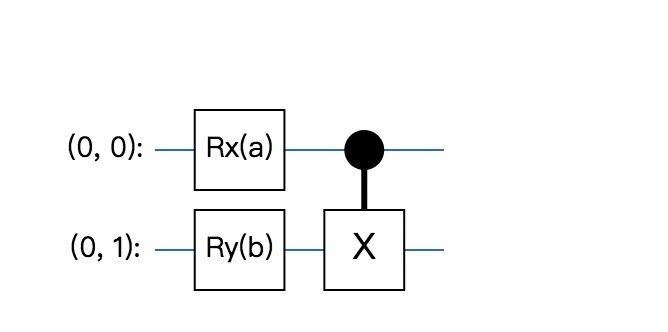# Calculate a state vector with a=0.5 and b=-0.5.resolver = cirq.ParamResolver({a: 0.5, b: -0.5})output_state_vector = cirq.Simulator().simulate(circuit, resolver).final_stateoutput_state_vector

数组（[0.9387913 + 0.j，-0.23971277 + 0.j，        0. + 0.06120872j，0. -0.23971277j]，dtype = complex64）

z0 = cirq.Z（q0）qubit_map = {q0：0，q1：1} z0.expectation_from_wavefunction（output_state_vector，qubit_map）.real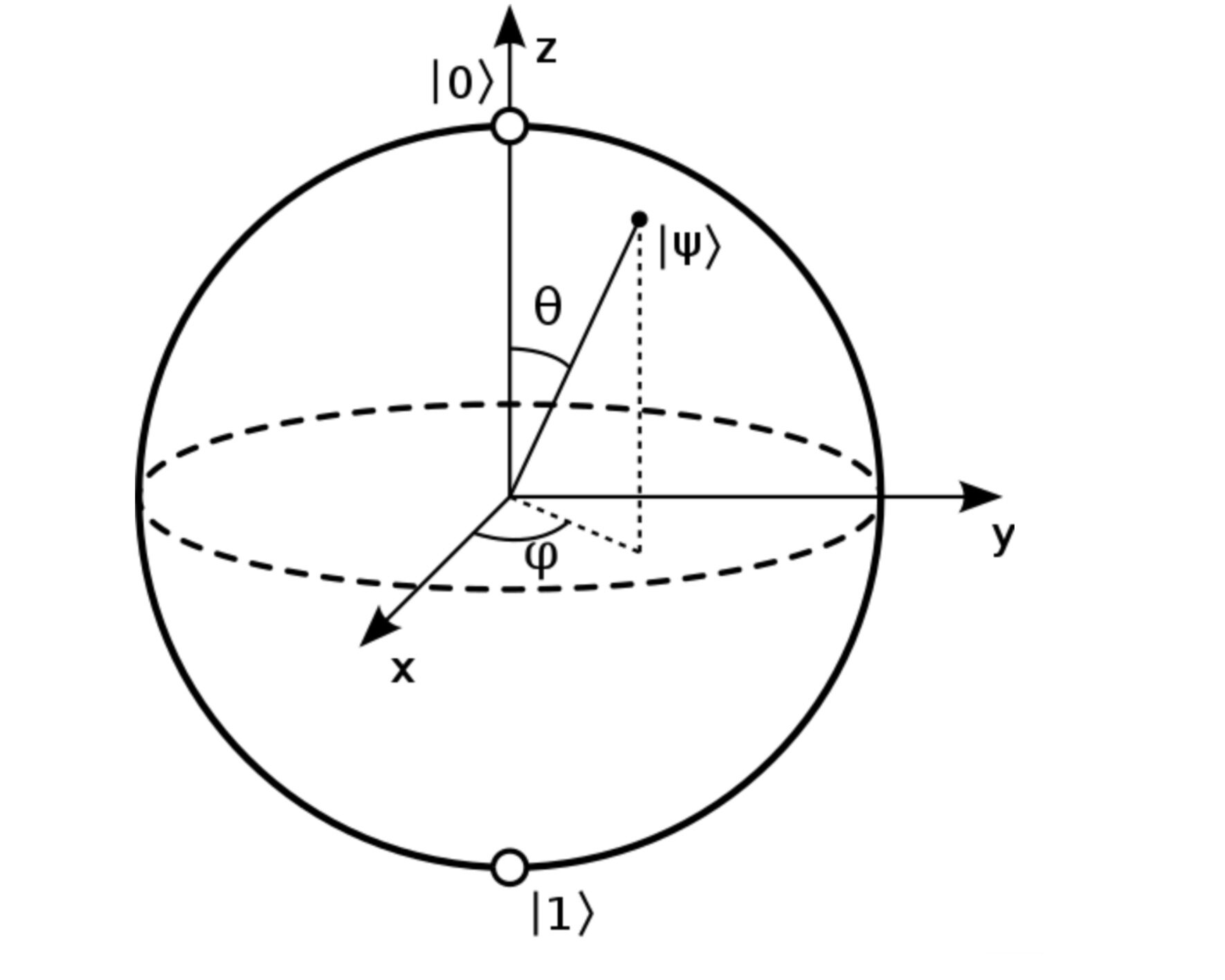0.8775825500488281

z0x1 = 0.5 * z0 + cirq.X(q1)z0x1.expectation_from_wavefunction(output_state_vector, qubit_map).real

-0.04063427448272705

### 量子电路作为张量

TensorFlow Quantum（TFQ）提供 tfq.convert_to_tensor 将 Cirq 对象转换为张量，开发者可以将 Cirq 对象发送到谷歌的 quantum layers 和 quantum ops，可以在 Cirq 电路和 Cirq Paulis 的列表或数组上调用该函数：

# Rank 1 tensor containing 1 circuit.circuit_tensor = tfq.convert_to_tensor([circuit])print(circuit_tensor.shape)print(circuit_tensor.dtype)

(1,)<dtype: 'string'>

# Rank 1 tensor containing 2 Pauli operators.pauli_tensor = tfq.convert_to_tensor([z0, z0x1])pauli_tensor.shape

TensorShape（）

### 量子经典混合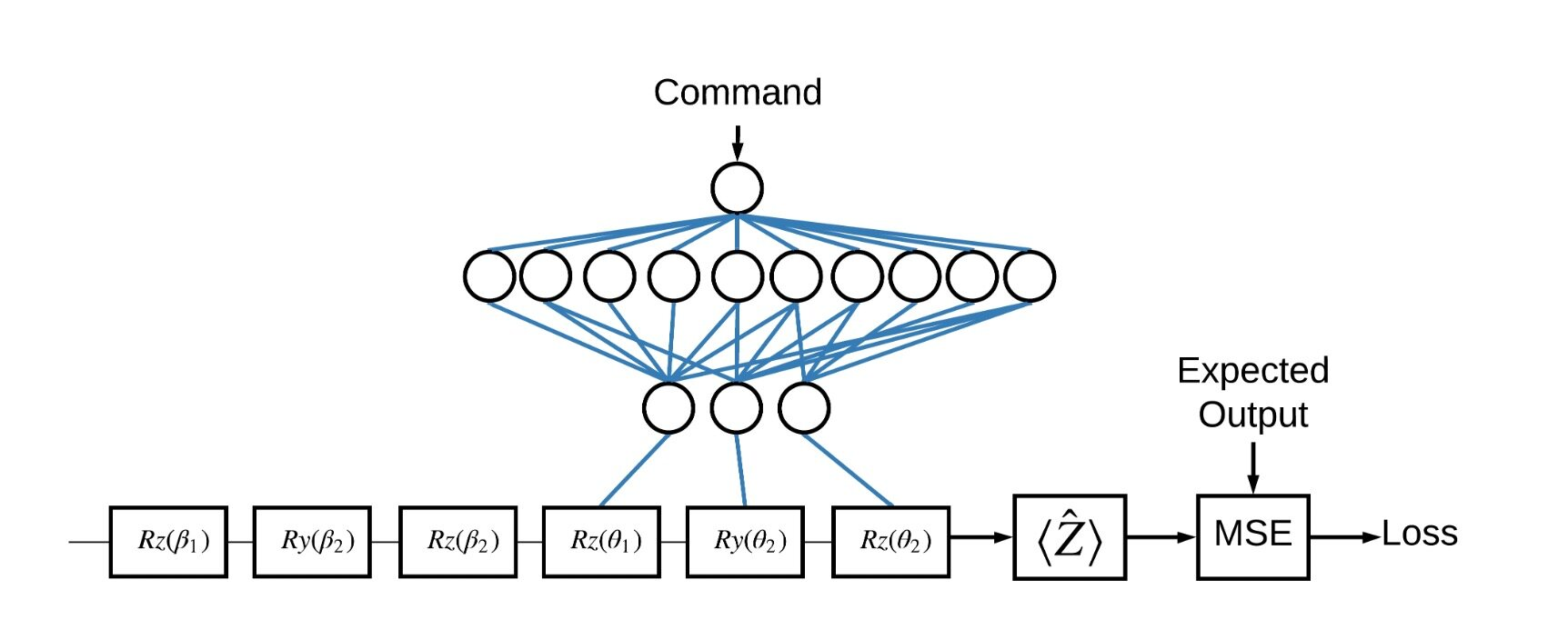# Parameters that the classical NN will feed values into.control_params = sympy.symbols('theta_1 theta_2 theta_3')# Create the parameterized circuit.qubit = cirq.GridQubit(0, 0)model_circuit = cirq.Circuit(    cirq.rz(control_params)(qubit),    cirq.ry(control_params)(qubit),    cirq.rx(control_params)(qubit))SVGCircuit(model_circuit)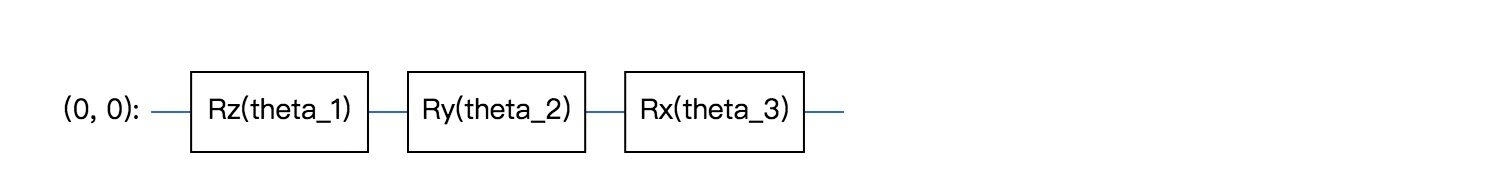# The classical neural network layers.controller = tf.keras.Sequential([    tf.keras.layers.Dense(10, activation='elu'),    tf.keras.layers.Dense(3)])

controller(tf.constant([[0.0],[1.0]])).numpy()

数组（[[0.，0.，0.]，       [-0.16003934，0.26334327，0.3790441]]，dtype = float32）

# This input is the simulated miscalibration that the model will learn to correct.circuits_input = tf.keras.Input(shape=(),                                # The circuit-tensor has dtype tf.string                                 dtype=tf.string,                                name='circuits_input')# Commands will be either 0 or 1, specifying the state to set the qubit to.commands_input = tf.keras.Input(shape=(1,),                                dtype=tf.dtypes.float32,                                name='commands_input')

dense_2 = controller(commands_input)# TFQ layer for classically controlled circuits.expectation_layer = tfq.layers.ControlledPQC(model_circuit,                                             # Observe Z                                             operators = cirq.Z(qubit))expectation = expectation_layer([circuits_input, dense_2])

# The full Keras model is built from our layers.model = tf.keras.Model(inputs=[circuits_input, commands_input],                       outputs=expectation)

tf.keras.utils.plot_model(model, show_shapes=True, dpi=70)

无法导入pydot。您必须安装pydot和graphviz才能使pydotprint运行。

# The command input values to the classical NN.commands = np.array([, ], dtype=np.float32)# The desired Z expectation value at output of quantum circuit.expected_outputs = np.array([, [-1]], dtype=np.float32)

random_rotations = np.random.uniform(0, 2 * np.pi, 3)noisy_preparation = cirq.Circuit(  cirq.rx(random_rotations)(qubit),  cirq.ry(random_rotations)(qubit),  cirq.rz(random_rotations)(qubit))datapoint_circuits = tfq.convert_to_tensor([  noisy_preparation] * 2)  # Make two copied of this circuit

datapoint_circuits.shape

TensorShape（）

model([datapoint_circuits, commands]).numpy()

数组（[[0.16693401]，       [-0.17766671]]，dtype = float32）

optimizer = tf.keras.optimizers.Adam(learning_rate=0.05)loss = tf.keras.losses.MeanSquaredError()model.compile(optimizer=optimizer, loss=loss)history = model.fit(x=[datapoint_circuits, commands],                    y=expected_outputs,                    epochs=30,                    verbose=0)

plt.plot(history.history['loss'])plt.title("Learning to Control a Qubit")plt.xlabel("Iterations")plt.ylabel("Error in Control")plt.show()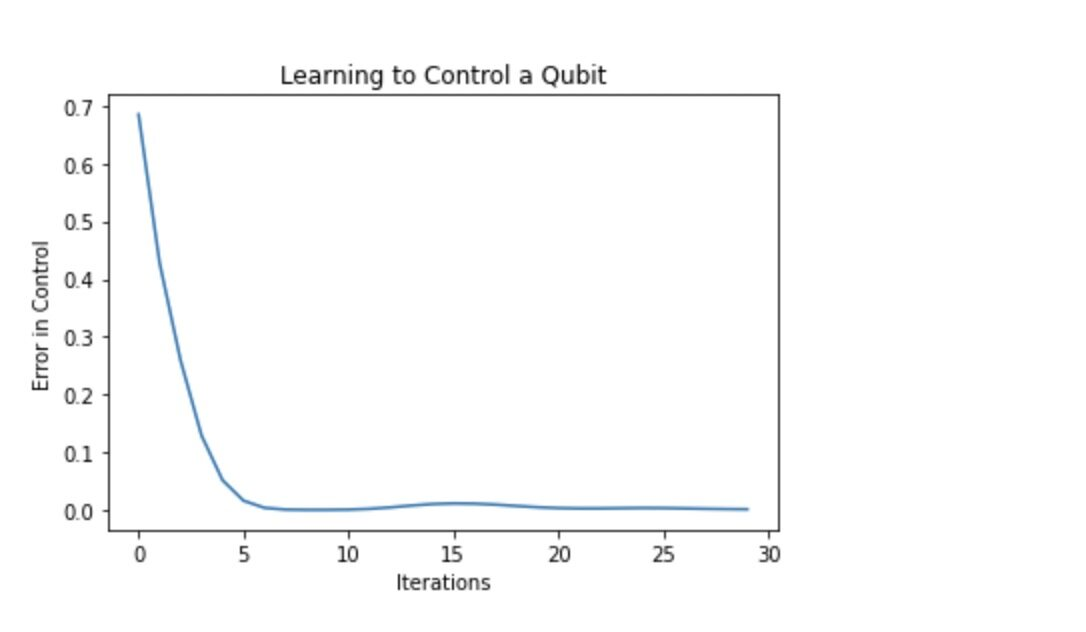def check_error(command_values, desired_values):  """Based on the value in command_value see how well you could prepare  the full circuit to have desired_value when taking expectation w.r.t. Z."""  params_to_prepare_output = controller(command_values).numpy()  full_circuit = noisy_preparation + model_circuit  # Test how well you can prepare a state to get expectation the expectation  # value in desired_values  for index in [0, 1]:    state = cirq_simulator.simulate(        full_circuit,        {s:v for (s,v) in zip(control_params, params_to_prepare_output[index])}    ).final_state    expectation = z0.expectation_from_wavefunction(state, {qubit: 0}).real    print(f'For a desired output (expectation) of {desired_values[index]} with'          f' noisy preparation, the controller\nnetwork found the following '          f'values for theta: {params_to_prepare_output[index]}\nWhich gives an'          f' actual expectation of: {expectation}\n')check_error(commands, expected_outputs)

For a desired output (expectation) of [1.] with noisy preparation, the controllernetwork found the following values for theta: [-0.22817115 -0.2512403  -1.5496594 ]Which gives an actual expectation of: 0.9663878679275513For a desired output (expectation) of [-1.] with noisy preparation, the controllernetwork found the following values for theta: [-1.2500848  1.4088008  2.5982447]Which gives an actual expectation of: -0.9780153036117554

model([datapoint_circuits, commands])

<tf.Tensor: shape=(2, 1), dtype=float32, numpy=array([[ 0.96638787],       [-0.9780154 ]], dtype=float32)>

Crip 项目地址：

2020-03-12 09:551866

## 评论

• ###### 构建贝叶斯深度学习分类器

最近贝叶斯深度学习变得越来越流行，并且正在开发新技术在模型中引入不确定性，同时参数量与传统模型相同。

• ###### 谷歌发布 Cirq：一个用于量子计算的 Python 开源库

Cirq旨在让嘈杂中型量子计算机（Noisy Intermediate Scale Quantum，NISQ）算法的编写、操作和优化变得更容易。Cirq还支持在本地模拟器上运行这些程序，并计划支持未来的量子硬件和量子云处理器。

• ###### 谷歌预发布新的量子处理器 Bristlecone

谷歌研究员Julian Kelly宣布了名为Bristlecone的新一代量子处理器，将量子处理器的量子比特提升到72个。

• ###### 训练速度提升超 3 倍！斯坦福推出全新深度学习并行计算框架 FlexFlow

斯坦福Matei团队提出全新深度学习并行计算框架FlexFlow

• ###### 谷歌发布第一代低温 CMOS 量子位控制器，功耗降低 1000 倍

该控制器在3开尔文环境下工作，功耗低于2毫瓦，尺寸仅为1毫米×1.6毫米

• ###### 深度学习基础：你打牢深度学习知识的地基了吗？

无论是单个神经元，还是结构非常复杂的深度学习网络，在推荐系统场景下，它们都是用来预测用户对某个物品的感兴趣程度的。

2020-09-28

• ###### 分布式机器学习平台大比拼：Spark、PMLS、TensorFlow、MXNet

文章从分布式系统的角度开展针对当前一些机器学习平台的研究，分析了这些平台在通信和控制上的瓶颈，并考虑了这些平台的容错性和易编程性。文章最后提供了一些结论性要点，并对分布式机器学习平台的未来研究工作提出了一些建议。

• ###### TensorFlow 这下更牛了，现已支持图神经网络

主要服务包括：建模 API、基于张量的图形数据结构、数据处理管道、实例模型

• ###### 微软对 macOS 和 Linux 开放量子开发工具集

继去年12月份对开发者发布量子开发工具集之后，微软宣布了它的第一次重要更新，包括支持macOS和Linux、与Python的互操作等。

• ###### 深度编解码：表示学习

今天我来和你分享由深度网络衍生出来的编解码结构，以及相关的表示学习概念。

2018-07-28

• ###### 基于机器学习的分子动力学模拟获得戈登·贝尔奖

2020年美国计算机协会（ACM）戈登·贝尔奖授予了来自美国和中国机构的研究团队，以表彰他们题为“用机器学习将分子动力学的从头计算方法的精度推至1亿个原子”的项目。

• ###### 人工智能初探：阿尔法狗是怎样的一只“狗”？

这节课是我们数据算法这章的最后一节课了，我们来聊聊到目前为止人工智能领域里的终极算法——深度学习算法。

2021-09-13

• ###### 量子标准化的里程碑：滑铁卢大学提出循环基准测试，评测量子系统性能

这种被称为“循环基准测试”的新方法，能够实现量子平台之间的性能比较。

• ###### 量子编程实践：Bell Pair 电路及 Deutsch 算法

QuTrunk是启科量子自主研发的量子编程框架。QuTrunk基于Python提供了量子编程API，对量子编程相关的基本概念做了代码层面的抽象封装和实现。本文将使用QuTrunk进行编程实践。

2022-11-04

• ###### 42 丨当我们谈深度学习的时候，我们都在谈什么？

数据挖掘十大经典算法属于传统的机器学习算法，机器学习算法和深度学习有什么关联呢？

2019-03-20

• ###### 最新研究称 AI 破解了薛定谔方程，量子化学取得重大突破

量子化学的这一根本性突破的潜在应用是巨大的。

• ###### 宣布推出开源时间序列建模工具包 Gluon Time Series

今天，我们宣布推出 Gluon Time Series (GluonTS)，这是一种使用 Gluon API 的 MXNet 时间序列分析工具包。

• ###### 层次化的神经网络：深度学习

今天我来和你分享深度神经网络一些主要的设计思想，以及看待深度神经网络的不同角度。

2018-07-26

• ###### 第 9 讲 | 深入区块链技术（一）：技术基础

区块链虽然是一个新兴的概念，但它依赖的技术一点也不新，如非对称加密技术、P2P 网络协议等。好比乐高积木，积木块是有限的，但是不同组合却能产生非常革新的事物。

2018-04-13

「架构实战营」

10月月更

#### Java开发究竟该如何学习，年末阿里百度等大厂技术面试题汇总，程序员翻身之路